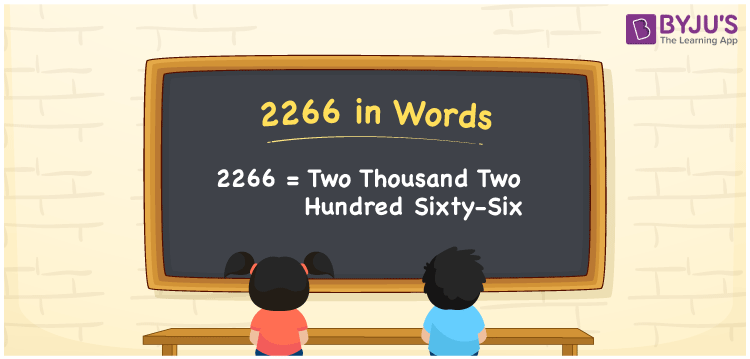# 2266 in Words

2266 in words is written as “two thousand two hundred sixty-six”. The number 2266 has four digits and it exists between the natural numbers 2265 and 2267. As we know, the number 2266 is also a cardinal number. For example, if you paid Rs. 2266 for books, it can be written as “I paid Rs. Two thousand two hundred sixty-six for books”.

 2266 in Words: Two Thousand Two Hundred Sixty-six. Two Thousand Two Hundred Sixty-six in Numerical Form: 2266.

## 2266 in English Words## How to Write 2266 in Words?

Go through the below place value table for the number 2266.

 Thousands Hundreds Tens Ones 2 2 6 6

The expanded form of 2266 is as follows:

= 2 × Thousand + 2 × Hundred + 6 × Ten + 6 × One

= 2 × 1000 + 2 × 100 + 6 × 10 + 6 × 1

= 2000 + 200 + 60 + 6

= 2266

= Two thousand two hundred sixty-six

Hence, 2266 in words is two thousand two hundred sixty-six.

2266 in words – Two thousand two hundred sixty-six

Is 2266 an odd number? – No

Is 2266 an even number? – Yes

Is 2266 a perfect square number? – No

Is 2266 a perfect cube number? – No

Is 2266 a prime number? – No

Is 2266 a composite number? – Yes

## Frequently Asked Questions on 2266 in Words

Q1

### Write 2266 in words.

2266 in words is two thousand two hundred sixty-six.

Q2

### Simplify 2200 + 66, and express it in words.

Simplifying 2200 + 66, we get 2266. Hence, 2266 in words is two thousand two hundred sixty-six.

Q3

### Is 2266 a composite number?

Yes, 2266 is a composite number.